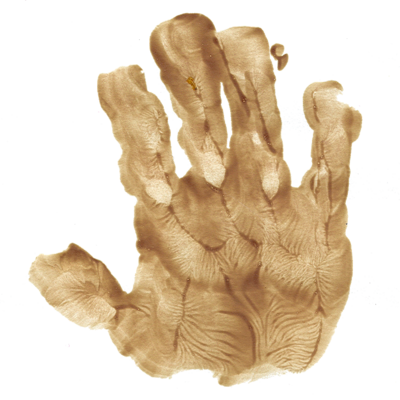I can calculate the motion of heavenly bodies, but not the madness of people.Isaac Newton, after a market collapse in 17th century England

It should be apparent that our Solar system is not a two-body system since the planets themselves exert gravitational forces on one another. Although the planetary forces are small in magnitude compared to the gravitational force of the Sun, they can still produce measureable effects. For example, the existence of the planets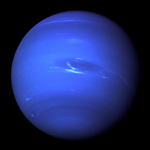Neptune and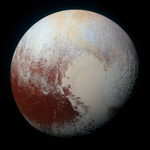Pluto was conjectured on the basis of a discrepancy between the experimentally measured orbit of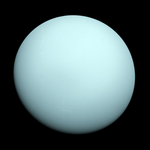Uranus and its predicted orbit that was calculated from known gravitational forces.

For simplicity, the model considered here is planar and not three-dimensional, and it is also assumed the force interaction is not strictly central since this is clearly not the case. The "AU" is an astronomical unit, which is roughly equal to 93 million miles.

## Planetary Perturbations

This program simulates a miniature Solar system of two planets about a fixed Sun-like star.
$\displaystyle{ \frac{\mathrm{d}^2 \mathbf{r}_1}{\mathrm{d} t^2} = -\frac{G M}{r_1^3} \mathbf{r}_1 + \frac{G m_2}{r_{21}^3} \mathbf{r}_{21} }$ $\displaystyle{ \frac{\mathrm{d}^2 \mathbf{r}_2}{\mathrm{d} t^2} = -\frac{G M}{r_2^3} \mathbf{r}_2 + \frac{G m_1}{r_{21}^3} \mathbf{r}_{21} }$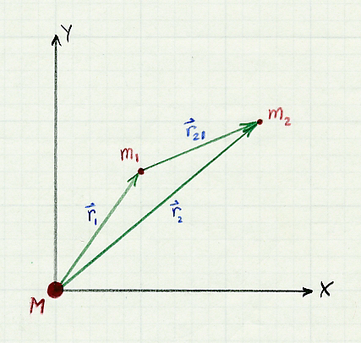Earth $x$-position: AU1 ≤ $x$ ≤ 5 AU ormi Earth $y$-velocity: AU/yr1 ≤ $v_y$ ≤ 5 AU/yr ormi/hr Pluto $x$-position: AU5 ≤ $x$ ≤ 10 AU ormi Pluto $y$-velocity: AU/yr1 ≤ $v_y$ ≤ 5 AU/yr ormi/hr Simulation time: yr2 ≤ $t$ ≤ 1000 yr orhr